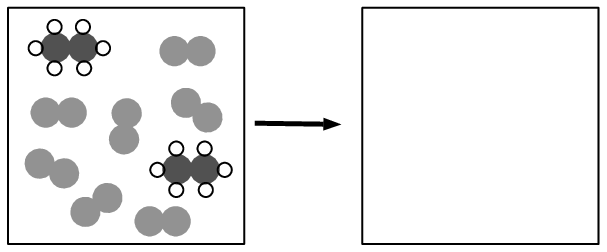Home Practice
For learners and parents For teachers and schools
Textbooks
Full catalogue
Pricing SupportLog in

We think you are located in United States. Is this correct?

# End of chapter exercises

## Representing chemical change

Textbook Exercise 14.4

Propane is a fuel that is commonly used as a heat source for engines and homes. Balance the following equation for the combustion of propane: $$\text{C}_{3}\text{H}_{8}\text{(l)} + \text{O}_{2}\text{(g)} \rightarrow \text{CO}_{2}\text{(g)} + \text{H}_{2}\text{O (g)}$$

Solution not yet available

Methane $$(\text{CH}_{4})$$ burns in oxygen according to the following reaction.

1. Complete the diagrams by drawing ball-and-stick models of the products.

2. Write a balanced chemical equation for the reaction and include state symbols.

Solution not yet available

Chemical weapons were banned by the Geneva Protocol in 1925. According to this protocol, all chemicals that release suffocating and poisonous gases are not to be used as weapons. White phosphorus, a very reactive allotrope of phosphorus, was recently used during a military attack. Phosphorus burns vigorously in oxygen. Many people got severe burns and some died as a result. The equation for this spontaneous reaction is: $$\text{P}_{4}\text{(s)} + \text{O}_{2}\text{(g)} \rightarrow \text{P}_{2}\text{O}_{5}\text{(s)}$$

1. Balance the chemical equation.

2. Prove that the law of conservation of mass is obeyed during this chemical reaction.

3. Name the product formed during this reaction.

4. Classify the reaction as a synthesis or decomposition reaction. Give a reason for your answer.

Solution not yet available

The following diagrams represent the combustion of ethane $$(\text{C}_{2}\text{H}_{6})$$. Complete the diagrams and write a balanced equation for the reaction. Indicate the state symbols.Solution not yet available

Balance the following chemical equation:

$\text{N}_{2}\text{O}_{5} \rightarrow \text{NO}_{2} + \text{O}_{2}$

Draw submicroscopic diagrams to represent this reaction.

Solution not yet available

Sulfur can be produced by the Claus process. This two-step process involves reacting hydrogen sulfide with oxygen and then reacting the sulfur dioxide that is produced with more hydrogen sulfide. The equations for these two reactions are:

\begin{align*} \text{H}_{2}\text{S} + \text{O}_{2} \rightarrow \text{SO}_{2} + \text{H}_{2}\text{O} \\ \text{H}_{2}\text{S} + \text{SO}_{2} \rightarrow \text{S} + \text{H}_{2}\text{O} \end{align*}

Balance these two equations.

Solution not yet available

Aspartame, an artificial sweetener, has the formula $$\text{C}_{14}\text{H}_{18}\text{N}_{2}\text{O}_{5}$$. Write the balanced equation for its combustion (reaction with $$\text{O}_{2}$$) to form $$\text{CO}_{2}$$ gas, liquid $$\text{H}_{2}\text{O}$$, and $$\text{N}_{2}$$ gas.

Solution not yet available